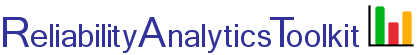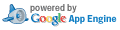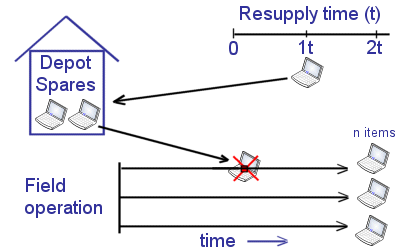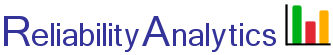# Spares Analysis

The Poisson distribution is useful for calculating the probability that a certain number of failures will occur over a certain length of time for equipment exhibiting a constant failure rate. If events are Poisson distributed, they occur at a constant average rate and the number of events occurring in any time interval is independent of the number of events occurring in any other time interval. Therefore, given an equipment population (n), the failure rate of each equipment (λ) and a time interval (t) during which no further spares will be available, the Poisson distribution can be used to predict the number of failures that will occur, with a certain probability (or confidence), and thus the number of depot spares required for time interval t. This calculation assumes that failed items cannot be repaired and put back into depot inventory during the resupply time interval, t.## Calculation Inputs:

1. Number of units to be supported (n):
2.
3. Time interval (t, hours):
4. Desired confidence (c):
5. Probability table:
6. Decimal places:

## Featured Reference:Asset Management Excellence: Optimizing Equipment Life-Cycle Decisions

Toolkit Home